These fun maths puzzles are a great way for second graders to dig into maths skills.

Click on a worksheet in the set below to see more info or download the PDF.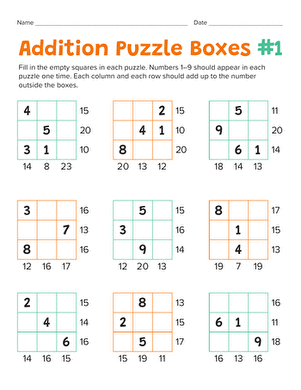Put your second grader's maths skills to the test with mathematical puzzle boxes. He'll practise doing addition facts in his head, and build logic skills.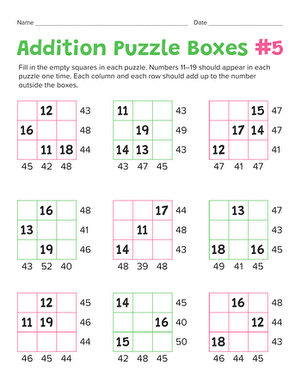Addition maths Puzzles #2

Your second grader will practise adding up to 50 while building her logic and problem solving skills with these maths puzzle boxes.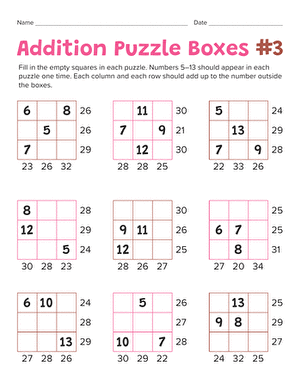Addition maths Puzzles #3

Put your child's addition abilities to the test with mathematical puzzle boxes. He'll practise his addition as he solves each puzzle.Addition maths Puzzles #4

Maths lovers can put their addition and subtraction skills to the test to figure out these Sudoku-style puzzles, a perfect introduction to basic algebra!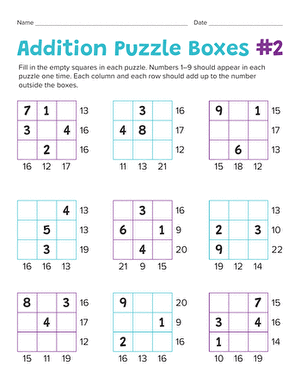Addition maths Puzzles #5

Make practising maths fun with maths puzzles. Your child will learn the basics of solving logic problems as he does maths in his head.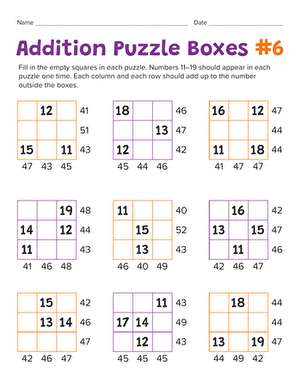Addition maths Puzzles #6

Add a fun twist to maths practise with these addition puzzles. Your maths maniac will get an introduction to logic and problem solving.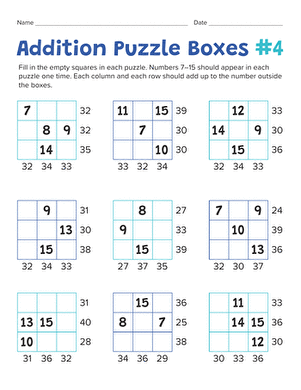Addition maths Puzzles #7

What better way to learn maths than with an addition puzzle? Your maths star will practise addition and get a taste of problem solving with logic.

Want to download this whole set as a single PDF?

Create new collection

0

New Collection>

0Items

What could we do to improve Education.com?

Please note: Use the Contact Us link at the bottom of our website for account-specific questions or issues.

What would make you love Education.com?